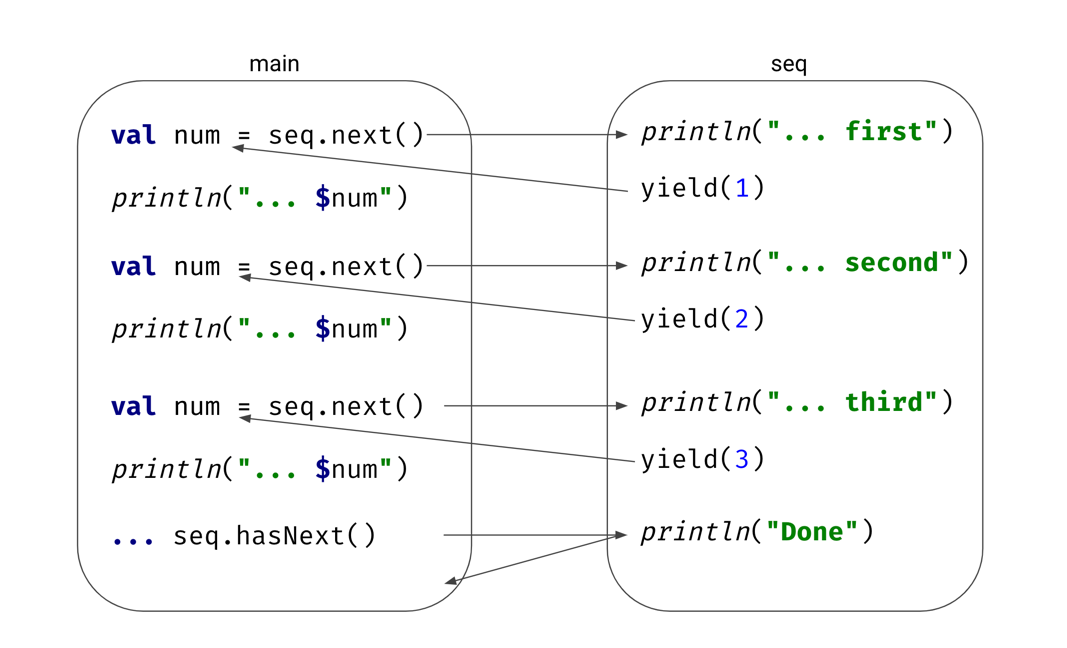# Sequence builders in Kotlin Coroutines

This is a chapter from the book Kotlin Coroutines. You can find Early Access on LeanPub.

In some other languages, like Python, Rust, or JavaScript, you can find structures that use some form of coroutines under the hood:

• async functions (also called async/await),
• generator functions (the functions, where next values are yielded).

We've already seen how async can be used in Kotlin, but it will be explained in detail in the chapter Coroutine builders. Instead of generators, Kotlin provides a sequence builder - a function used to create a sequence1.

A Kotlin sequence is a similar concept to a collection (like List or Set), but it is evaluated lazily. Meaning, the next element is always calculated on-demand, when it is needed. As a result, sequences:

• do the minimal number of required operations,
• can be infinite,
• are more memory-efficient2.

Due to those characteristics, it makes a lot of sense to define a builder, where next elements are calculated and "yielded" on demand. We define it using function sequence. Inside its lambda expression, we can call the yield function, to produce next elements of this sequence.

import kotlin.* //sampleStart val seq = sequence { yield(1) yield(2) yield(3) } fun main() { for (num in seq) { print(num) } // 123 } //sampleEnd

sequence function here is a small DSL. Its argument is a lambda expression with a receiver (suspend SequenceScope<T>.() -> Unit). Inside it, the receiver this refers to an object of type SequenceScope<T>. It has functions like yield. When we call yield(1), it is equivalent to call this.yield(1), because this can be used implicitly. If this is your first contact with lambda expressions with receivers, I recommend starting from learning about them and about DSL creation, as they are used intensively in Kotlin Coroutines.

What is essential here, is that each number is generated on demand, not in advance. You could observe this process well if we print something on both the builder, and on the place where we handle our sequence.

import kotlin.* //sampleStart val seq = sequence { println("Generating first") yield(1) println("Generating second") yield(2) println("Generating third") yield(3) println("Done") } fun main() { for (num in seq) { println("Next number is $num") } } // Generating first // Next number is 1 // Generating second // Next number is 2 // Generating third // Next number is 3 // Done //sampleEnd Let's analyze how it works. We ask for the first number, so we enter the builder. We print "Generating first", and we yield number 1. Then it is handled, and so "Next number is 1" is printed. Then something crucial happens - we jump with execution to the place where we previously stopped, to find another number. This would be impossible without a suspension mechanism, as it wouldn't be possible to stop a function in the middle, and resume it from the same point later in the future. Thanks to suspension, we can do this, we can freely jump with execution between main and the sequence generator.When we ask for the next value in the sequence, we resume in the builder straight after the previous yield. To see it more clearly, let's manually ask for a few values from the sequence. import kotlin.* //sampleStart val seq = sequence { println("Generating first") yield(1) println("Generating second") yield(2) println("Generating third") yield(3) println("Done") } fun main() { val iterator = seq.iterator() println("Starting") val first = iterator.next() println("First:$first") val second = iterator.next() println("Second: \$second") // ... } // Prints: // Starting // Generating first // First: 1 // Generating second // Second: 2 //sampleEnd

Here we used an iterator to get the next values. At any point, we can call it again, to jump in the middle of the builder function, and generate the next value. Would it be possible without coroutines? Maybe, if we would dedicate a thread for that. Such thread would need to be maintained, and that would be a huge cost. With coroutines, it is fast and simple. Moreover, we can keep this iterator for as long as we wish to as it costs nearly nothing. Soon we will learn how this mechanism works under the hood (in the chapter Suspension under the hood).

### Real-life usages

There are a few use-cases where sequence builders are used. The most typical one is generating a mathematical sequence, like a Fibonacci sequence.

import java.math.BigInteger //sampleStart val fibonacci: Sequence<BigInteger> = sequence { var first = 0.toBigInteger() var second = 1.toBigInteger() while (true) { yield(first) val temp = first first += second second = temp } } fun main() { print(fibonacci.take(10).toList()) } // [0, 1, 1, 2, 3, 5, 8, 13, 21, 34] //sampleEnd

This builder can also be seen used for generating random numbers or texts.

fun randomNumbers( seed: Long = System.currentTimeMillis() ): Sequence<Int> = sequence { val random = Random(seed) while (true) { yield(random.nextInt()) } } fun randomUniqueStrings( length: Int, seed: Long = System.currentTimeMillis() ): Sequence<String> = sequence { val random = Random(seed) val charPool = ('a'..'z') + ('A'..'Z') + ('0'..'9') while (true) { val randomString = (1..length) .map { i -> random.nextInt(charPool.size) } .map(charPool::get) .joinToString(""); yield(randomString) } }.distinct()

Sequence builder should not be used for suspending operations other than yielding. If you need, for instance, to fetch data, we rather use Flow, that will be explained later in the book. The way its builder works is similar to the sequence builder, but Flow has support for other coroutine features.

fun allUsersFlow( api: UserApi ): Flow<User> = flow { var page = 0 do { val users = api.takePage(page++) // suspending emitAll(users) } while (!users.isNullOrEmpty()) }

We've learned about the sequence builder, and why it needs suspension to work correctly. We've seen suspension in action, it is time to dive even deeper to understand how suspension works when we use it directly.

1:

Even better, it offers flow builders. Flow is a similar, but much more powerful concept, which we will explain later in the book.